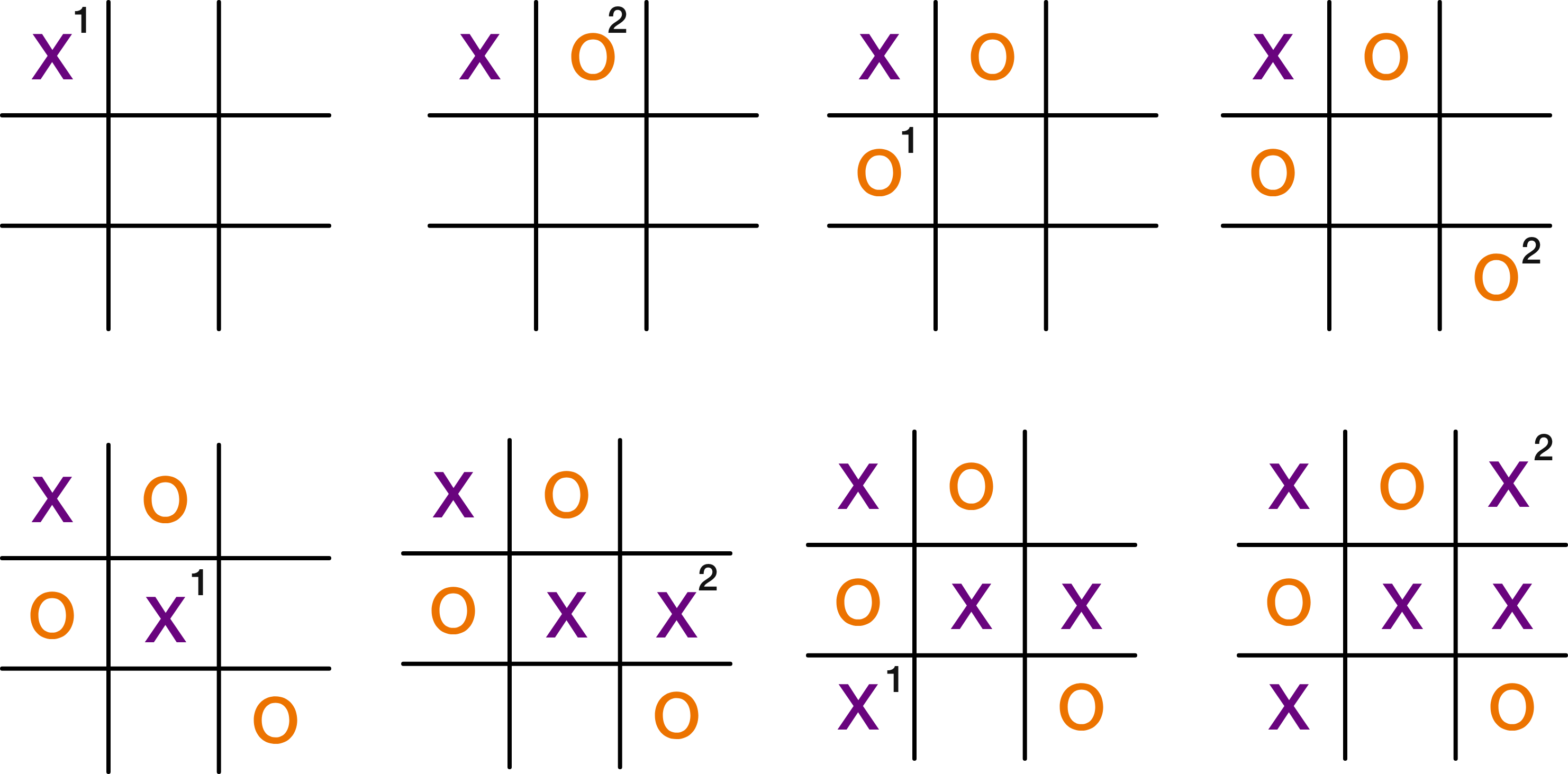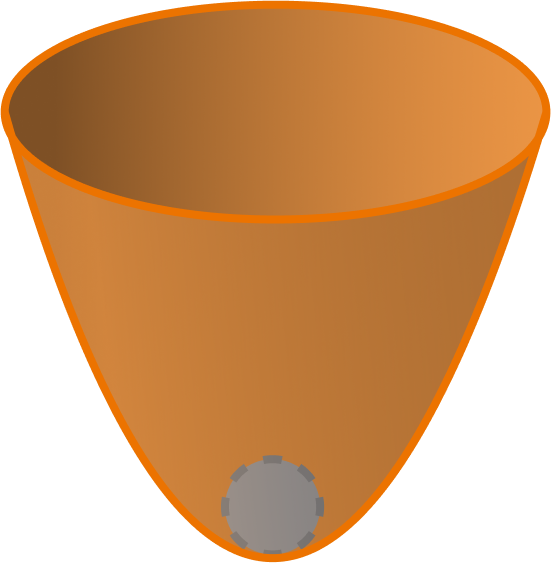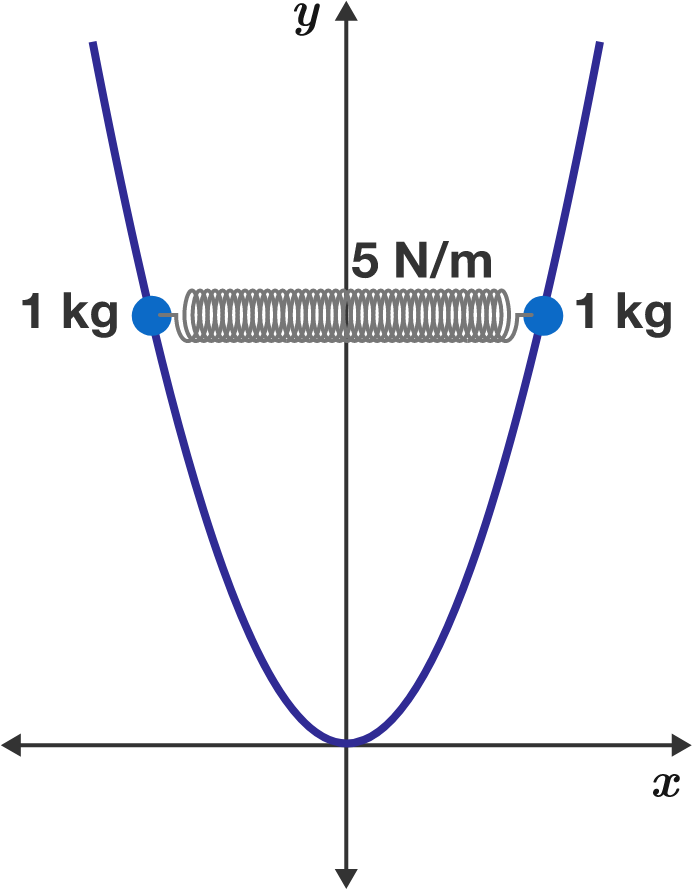# Problems of the Week

Contribute a problem

# 2018-05-14 Intermediate

Wild tic-tac-toe is like traditional tic-tac-toe except that on each turn, a player may place an X or an O. The first person to get a row, column, or diagonal of three Xs or Os wins the game.

If Wild tic-tac-toe is played optimally, who should win?This particular game ends on victory for player 2. The moves (and which player made them) are marked.

\begin{aligned} 1+1 \times 2 \times 3 \times 4 &= 5^2 \\ 1+2 \times 3 \times 4 \times 5 &= 11^2 \\ 1 + 3 \times 4 \times 5 \times 6 &= 19^2 \end{aligned}

Is 1 plus the product of four consecutive integers always a perfect square?This bowl is in the shape of the paraboloid $z=x^2+y^2$. A ball falls to the bottom such that it touches the lowest point $(0,0,0)$ of the bowl.

What is the maximum possible radius of the ball?Two $\SI{1}{\kilo\gram}$ beads are at the ends of a compressed spring resting on a smooth parabolic wire $\big(y = x^2\big).$ The spring has a natural length of $\SI{1}{\meter}$ and a force constant of $\SI[per-mode=symbol]{5}{\newton\per\meter}.$

If the system is in static equilibrium, how far away from the $y$-axis is each bead (in meters)?

Note: The spring is horizontal and gravity is $\SI[per-mode=symbol]{10}{\meter\per\second\squared}$ in the $-y$ direction.

$012345678910{\color{#3D99F6}111}21314\ldots$

The above is the beginning of all non-negative integers concatenated into a single infinite string.

$\color{#3D99F6}111$ is the first sub-string with 3 identical digits in a row, with different digits preceding and following the run of the identical digits. The first $\color{#3D99F6}1$ of $\color{#3D99F6}111$ comes at position 13 (from left) in the infinite string.

Where is the first such sub-string with 4 identical digits?

Give the position of the first digit of the four.

×